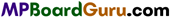These MP Board Class 11th Chemistry Notes for Chapter 1 Some Basic Concepts of Chemistry help students to get a brief overview of all the concepts.

## MP Board Class 11th Chemistry Notes Chapter 1 Some Basic Concepts of Chemistry

→ Laws of chemical combinations :

1. Law of conservation of matter : In a chemical change, total mass of reactants and total mass of products are the same.
2. Law of constant proportion : Ratio by weight of constituent elements in a chemical compound remains always the same.
3. Law of multiple proportion : When an element of definite weight combines with an other element of definite weight, two or more than two compounds are formed in a simple ratio.
4. Law of equivalence proportion : When two elements combine with a definite mass of an other element separately, the weight of both elements will be in the ratio in which they combine with each other or is simple multiple of that.
5. Gay Lussac’s law : When gases react, their volumes are in simple ratio and volume of products ifgaseous are also in simple ratio of reacting gases.

→ Atomic mass : Atomic mass of an element is the number which shows that one atom of element is how many times heavier than that of 1/12th part of C12.→ Molecular mass: Molecular mass of an element is the number which shows that one molecule of a compound is how many times heavier than 1/12 th part of C12 atom.
Molecular mass = 2 × Vapour density.

→ Mole : It shows collection of particles of a substance. In one mole of substance (molecule, atom or ion), number of particles are 6023 × 1023. It is also called as Avogadro’s number.

→ Volume of 1 mole of.a gas at N.T.R is 224 litre.

→ Percentage : Number of grams of solute present in 100 gm of a solution.

→ Molarity : No. of moles of solute in a litre of solution.

→ Percentage composition : Number of parts of each element present in 100 parts according to mass in a compound.

→ Chemical formula: Representation of molecule of a compound in terms of symbol of elements.
It is of three types :

1. Empirical formula: Formula which gives simple whole number ratio of different elements.
2. Molecular formula : Formula which gives exact number of different atoms present in a molecule.
3. Structural formula : Represents the arrangement of various atoms present in molecule of compound.→ Chemical Equation : The short representation of a chemical reaction in terms of symbols and formula of reactants and products.

→ Element: Substance made up of only one kind of atoms.

→ Compound : Compound is formed by the combination of two or more elements in a definite ratio.

→ Mixture : Mixture is formed by mixing two or more substances in any ratio.

→ There are seven basic units of measurement. All other units are derived from these.

→ Limiting reactants are those which are present in less amount in chemical reaction.

→ Total number of digits in a number are called significant figures.

→ Some useful conversion fractions :

1 pm = 10-12m, 1Å = 10-10m, 1 nm= 10-9 m
1L = 10-3 m3 = 1 dm3, 760 torr = 101325 Pa = Nm-2.
1 atm = 1.01325 bar
1 bar =105 Nm-2 = 105 Pa, 1 atm = 760mm Hg = 760 torr 1 calorie =4184 joule, 1 Electron volt = 1-6022 x 10-19 joule
Avogadro Number (N0) = 6 022 x 1023 (Atom, Molecule, ion or electron)
1 Atomic mass unit (amu or u) = $$\frac{\text { Atomic mass }}{\text { Avogadro Number }}=\frac{A}{N_{0}}$$→ Normality (N) : Number of gram equivalent of solute per litre of solution.

→ Molality (m) : Number of moles of solute present in 1 kg solvent of the solution.

→ Mole Fraction : Ratio of number of moles of a component to the total number of moles of all the components of the solution.
In a mixture of solution number of moles of components 1 and 2 are n1and n2.
Mole fraction of component 1 will be
X1 = X1 + X2 = 1
Thus X1 = 1 – X2

→ Average mole mass of an element ($$\bar{M}$$)
= $$\frac{\text { Sum of mole mass of Isotopes } \times \% \text { amount (abundance) }}{100}$$
Sum of mole mass of Isotopes X % amount (abundance)
or $$\bar{M}$$ = Σfi × Ai
Where fi = Fraction of amount of Isotope
Ai = Sum of masses of Isotopes.